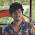## Thursday, October 23, 2008

### Mind-twisting Mathematics Questions

How good is your math? Are you up for a challenge? Try solving these riddles.

"The sign of a good maths puzzle is one where you haven't a
clue where to begin searching for a solution - one which at first sight looks a
nightmare, and with which a further look drives one to despair" - Mr Know-it-all (2001)

Below were questions prepared and designed by me. If you can't answer the questions, please don't fire at me, huh…Wassup, don't show me your sour face, smile a bit, will you?

Well, I've worked out these questions for certain days. Now, it's your turn to have your mind twisted while trying to solve these Mathematical questions. I assure you that these questions will keep your brain staying active to delay aging process. Regardless your ages, occupations, and qualifications, you're invited to challenge yourself with these trickiest questions. Do tell me your score, por favor (“please” in Spanish)!

Now, get yourself ready, sit still, and here we go…Good luck!
Mathematics Questions

1. Given that 1 = 5, 2 = 15, 3 = 125, 4 = 1235, find the value of 5
2. James needs 15 days to assembly bulks of accessories for a car, but Dave needs only 12 days to accomplish the task. Now, let me ask you how much more pieces of accessories does Dave manage to accomplish as compared to James?
3. Given that a + b = c = 10. Now, find the value of 2a + a + 3b.
4. How to get 24 from the four whole numbers of 10,10, 10 and 3? You can apply addition, subtraction, multiplication and division to get the answer
5. How to get 10 from the four whole numbers of 1,1,9 and 9?
6. What's the proper way to calculate 8-1-3x/2 = 0?
7. Calculate the value of 4x³-2x²-3x+1=0
8. Try to simplify this equation (10-2X)*(6-2X )*X in its mathematical form
9. There're 16 small squares in one big square. How many squares are they in total?10.

Without using calculator, calculate 9,9,9 and 1 to get 100. You can apply addition, subtraction, multiplication and division to get the answer.
11.

Now, challenge yourself by using addition, subtraction, division or multiplication to calculate 1,3,4, and 6 to obtain 24
12.

Again, using addition, subtraction, division or multiplication, calculate the whole numbers of 5,6,2 and 9 to obtain 11
13.

How do you obtain 100 with 9,9,9 and 9? You can arrange the 9 digit in the position as you like
14.

How do you obtain 24 with digit numbers of 5, 5, 5 and 1?
15.

How do you obtain 24 with digit numbers of 3,3,8 and 8?
16.

Using addition, subtraction, division and multiplication, find the answer of 21 from the digit numbers of 5,5,5,5 and 5?

Note: Each question earns you 3 marks.

Answer Here

#### 2 comments:

1.This comment has been removed by the author.

2.Tougest and challenging question
Two people walks at spped of 2km/hr and 4km/hr respectively at same direction.A train moving in the same direction crosses the first person in 9 sec and second person in 10 sec.Find the speed of the train and length of the train

Pls sent answer to jaideepjm@gmail.com as quick as possible